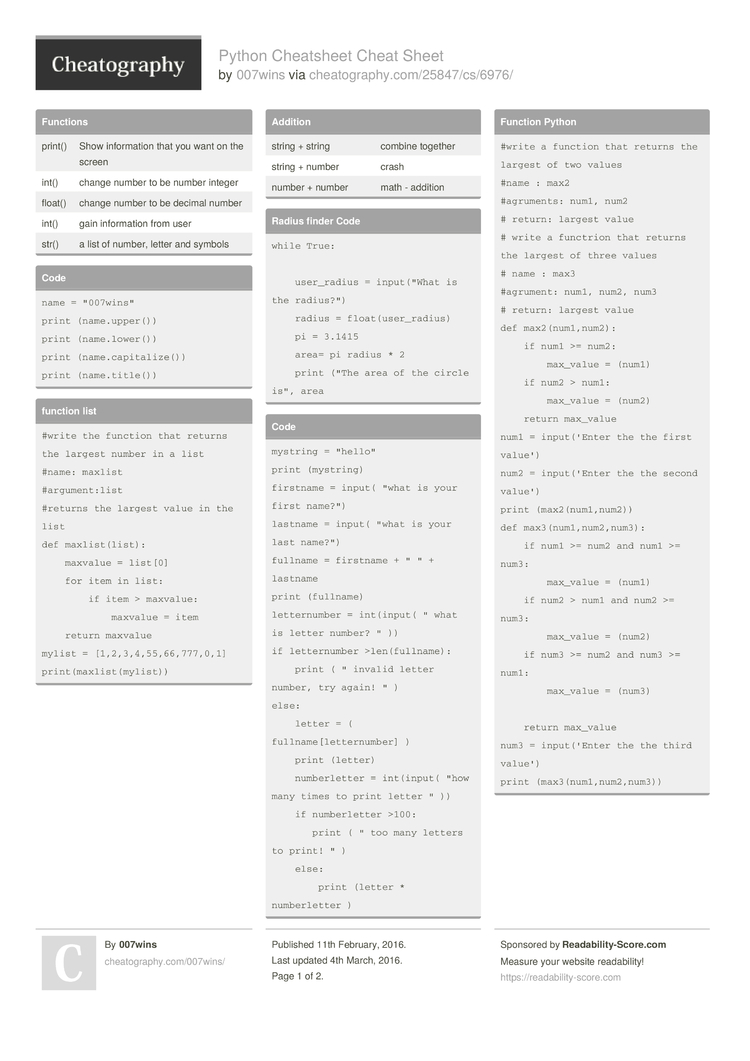# Python Cheatsheet Cheat Sheet by 007wins

Vocabulary

### Functions

 print() Show inform­­ation that you want on the screen int() change number to be number integer float() change number to be decimal number int() gain inform­­ation from user str() a list of number, letter and symbols

### Code

 ```name = "007wins" print (name.upper()) print (name.lower()) print (name.capitalize()) print (name.title())```

### function list

 ```#write the function that returns the largest number in a list #name: maxlist #argument:list #returns the largest value in the list def maxlist(list):     maxvalue = list     for item in list:         if item > maxvalue:             maxvalue = item     return maxvalue mylist = [1,2,3,4,55,66,777,0,1] print(maxlist(mylist))```

 string + string combine together string + number crash number + number math - addition

 ```while True:          user_radius = input("What is the radius?")     radius = float(user_radius)     pi = 3.1415     area= pi radius * 2     print ("The area of the circle is", area```

### Code

 ```mystring = "hello" print (mystring) firstname = input( "what is your first name?") lastname = input( "what is your last name?") fullname = firstname + " " + lastname print (fullname) letternumber = int(input( " what is letter number? " )) if letternumber >len(fullname):     print ( " invalid letter number, try again! " ) else:     letter = ( fullname[letternumber] )     print (letter)     numberletter = int(input( "how many times to print letter " ))     if numberletter >100:        print ( " too many letters to print! " )     else:         print (letter * numberletter )```

### Function Python

 ```#write a function that returns the largest of two values #name : max2 #agruments: num1, num2 # return: largest value # write a functrion that returns the largest of three values # name : max3 #agrument: num1, num2, num3 # return: largest value def max2(num1,num2):     if num1 >= num2:         max_value = (num1)     if num2 > num1:         max_value = (num2)     return max_value num1 = input('Enter the the first value') num2 = input('Enter the the second value') print (max2(num1,num2)) def max3(num1,num2,num3):     if num1 >= num2 and num1 >= num3:         max_value = (num1)     if num2 > num1 and num2 >= num3:         max_value = (num2)     if num3 >= num2 and num3 >= num1:         max_value = (num3)              return max_value num3 = input('Enter the the third value') print (max3(num1,num2,num3))```

### Multip­­li­c­ation and Exponents

 string * number combine that string string* string crash number * number math - multiply string ** string crash number ** number math - exponent string ** number crash

### Math

 == equal to != no equal to < less than > more than <= less than or equal to >= more than or equal to

### Vocabulary

 variable hold a value and can be change string a list of character such as number, letter and symbols integer number whole number or counting number float number the number in decimal syntax grammar or structure of lauguage value the number or string can be store in valuable loop mudole the text for storing for python code blank input gain inform­ation form User comment print To show inform­ation on code code syntax error make impossible to the parse2 Pages
//media.cheatography.com/storage/thumb/007wins_python-cheatsheet.750.jpg

PDF (recommended)